# 11 Important Model Evaluation Metrics for Machine Learning Everyone should know

## Overview

• Evaluating a model is a core part of building an effective machine learning model
• There are several evaluation metrics, like confusion matrix, cross-validation, AUC-ROC curve, etc.
• Different evaluation metrics are used for different kinds of problems

This article was originally published in February 2016 and updated in August 2019. with four new evaluation metrics.

## Introduction

The idea of building machine learning models works on a constructive feedback principle. You build a model, get feedback from metrics, make improvements and continue until you achieve a desirable accuracy. Evaluation metrics explain the performance of a model. An important aspect of evaluation metrics is their capability to discriminate among model results.

I have seen plenty of analysts and aspiring data scientists not even bothering to check how robust their model is. Once they are finished building a model, they hurriedly map predicted values on unseen data. This is an incorrect approach.

Simply building a predictive model is not your motive. It’s about creating and selecting a model which gives high accuracy on out of sample data. Hence, it is crucial to check the accuracy of your model prior to computing predicted values.In our industry, we consider different kinds of metrics to evaluate our models. The choice of metric completely depends on the type of model and the implementation plan of the model.

After you are finished building your model, these 11 metrics will help you in evaluating your model’s accuracy. Considering the rising popularity and importance of cross-validation, I’ve also mentioned its principles in this article.

And if you’re starting out your machine learning journey, you should check out the comprehensive and popular ‘Applied Machine Learning’ course which covers this concept in a lot of detail along with the various algorithms and components of machine learning.

1. Confusion Matrix
2. F1 Score
3. Gain and Lift Charts
4. Kolmogorov Smirnov Chart
5. AUC – ROC
6. Log Loss
7. Gini Coefficient
8. Concordant – Discordant Ratio
9. Root Mean Squared Error
10. Cross Validation (Not a metric though!)

## Warming up: Types of Predictive models

When we talk about predictive models, we are talking either about a regression model (continuous output) or a classification model (nominal or binary output). The evaluation metrics used in each of these models are different.

In classification problems, we use two types of algorithms (dependent on the kind of output it creates):

1. Class output: Algorithms like SVM and KNN create a class output. For instance, in a binary classification problem, the outputs will be either 0 or 1. However, today we have algorithms which can convert these class outputs to probability. But these algorithms are not well accepted by the statistics community.
2. Probability output: Algorithms like Logistic Regression, Random Forest, Gradient Boosting, Adaboost etc. give probability outputs. Converting probability outputs to class output is just a matter of creating a threshold probability.

In regression problems, we do not have such inconsistencies in output. The output is always continuous in nature and requires no further treatment.

Illustrative Example

For a classification model evaluation metric discussion, I have used my predictions for the problem BCI challenge on Kaggle. The solution of the problem is out of the scope of our discussion here. However the final predictions on the training set have been used for this article. The predictions made for this problem were probability outputs which have been converted to class outputs assuming a threshold of 0.5.

## 1. Confusion Matrix

A confusion matrix is an N X N matrix, where N is the number of classes being predicted. For the problem in hand, we have N=2, and hence we get a 2 X 2 matrix. Here are a few definitions, you need to remember for a confusion matrix :

• Accuracy : the proportion of the total number of predictions that were correct.
• Positive Predictive Value or Precision : the proportion of positive cases that were correctly identified.
• Negative Predictive Value : the proportion of negative cases that were correctly identified.
• Sensitivity or Recall : the proportion of actual positive cases which are correctly identified.
• Specificity : the proportion of actual negative cases which are correctly identified.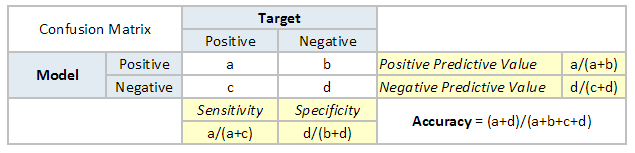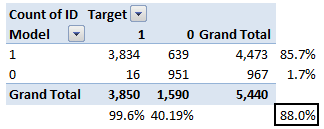The accuracy for the problem in hand comes out to be 88%.  As you can see from the above two tables, the Positive predictive Value is high, but negative predictive value is quite low. Same holds for Sensitivity and Specificity. This is primarily driven by the threshold value we have chosen. If we decrease our threshold value, the two pairs of starkly different numbers will come closer.

In general we are concerned with one of the above defined metric. For instance, in a pharmaceutical company, they will be more concerned with minimal wrong positive diagnosis. Hence, they will be more concerned about high Specificity. On the other hand an attrition model will be more concerned with Sensitivity. Confusion matrix are generally used only with class output models.

## 2. F1 Score

In the last section, we discussed precision and recall for classification problems and also highlighted the importance of choosing precision/recall basis our use case. What if for a use case, we are trying to get the best precision and recall at the same time? F1-Score is the harmonic mean of precision and recall values for a classification problem. The formula for F1-Score is as follows: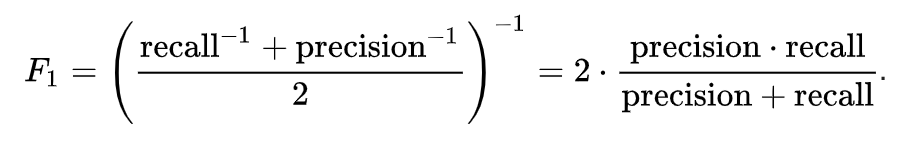Now, an obvious question that comes to mind is why are taking a harmonic mean and not an arithmetic mean. This is because HM punishes extreme values more. Let us understand this with an example. We have a binary classification model with the following results:

Precision: 0, Recall: 1

Here, if we take the arithmetic mean, we get 0.5. It is clear that the above result comes from a dumb classifier which just ignores the input and just predicts one of the classes as output. Now, if we were to take HM, we will get 0 which is accurate as this model is useless for all purposes.

This seems simple. There are situations however for which a data scientist would like to give a percentage more importance/weight to either precision or recall. Altering the above expression a bit such that we can include an adjustable parameter beta for this purpose, we get: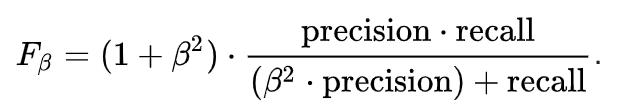Fbeta measures the effectiveness of a model with respect to a user who attaches β times as much importance to recall as precision.

## 3. Gain and Lift charts

Gain and Lift chart are mainly concerned to check the rank ordering of the probabilities. Here are the steps to build a Lift/Gain chart:

Step 1 : Calculate probability for each observation

Step 2 : Rank these probabilities in decreasing order.

Step 3 : Build deciles with each group having almost 10% of the observations.

Step 4 : Calculate the response rate at each deciles for Good (Responders) ,Bad (Non-responders) and total.

You will get following table from which you need to plot Gain/Lift charts: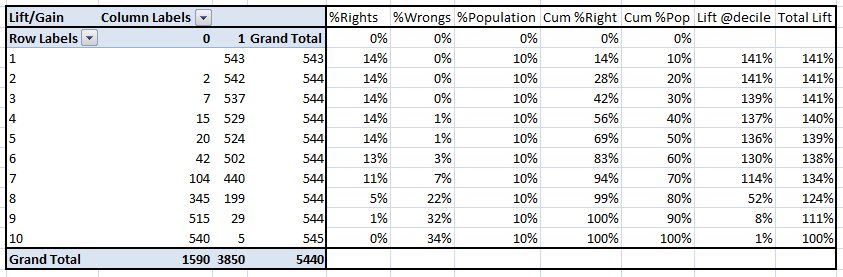This is a very informative table. Cumulative Gain chart is the graph between Cumulative %Right and Cummulative %Population. For the case in hand here is the graph :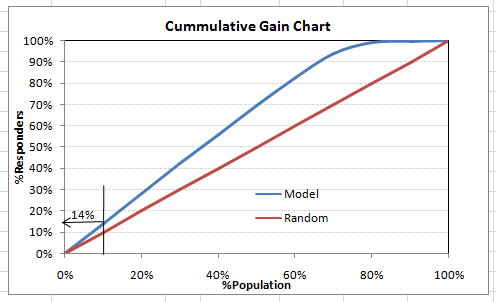This graph tells you how well is your model segregating responders from non-responders. For example, the first decile however has 10% of the population, has 14% of responders. This means we have a 140% lift at first decile.

What is the maximum lift we could have reached in first decile? From the first table of this article, we know that the total number of responders are 3850. Also the first decile will contains 543 observations. Hence, the maximum lift at first decile could have been 543/3850 ~ 14.1%. Hence, we are quite close to perfection with this model.

Let’s now plot the lift curve. Lift curve is the plot between total lift and %population. Note that for a random model, this always stays flat at 100%. Here is the plot for the case in hand :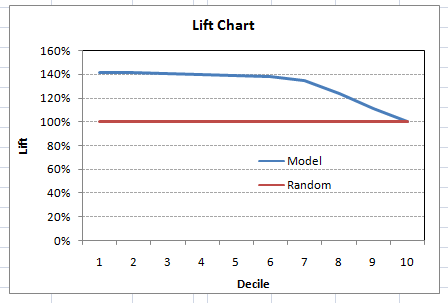You can also plot decile wise lift with decile number :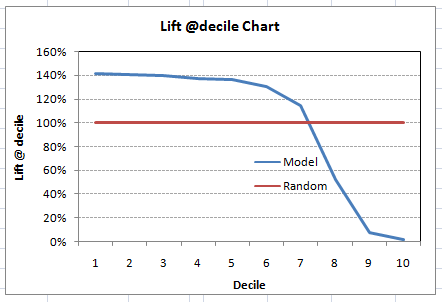What does this graph tell you? It tells you that our model does well till the 7th decile. Post which every decile will be skewed towards non-responders. Any model with lift @ decile above 100% till minimum 3rd decile and maximum 7th decile is a good model. Else you might consider over sampling first.

Lift / Gain charts are widely used in campaign targeting problems. This tells us till which decile can we target customers for an specific campaign. Also, it tells you how much response do you expect from the new target base.

## 4. Kolomogorov Smirnov chart

K-S or Kolmogorov-Smirnov chart measures performance of classification models. More accurately, K-S is a measure of the degree of separation between the positive and negative distributions. The K-S is 100, if the scores partition the population into two separate groups in which one group contains all the positives and the other all the negatives.

On the other hand, If the model cannot differentiate between positives and negatives, then it is as if the model selects cases randomly from the population. The K-S would be 0. In most classification models the K-S will fall between 0 and 100, and that the higher the value the better the model is at separating the positive from negative cases.

For the case in hand, following is the table :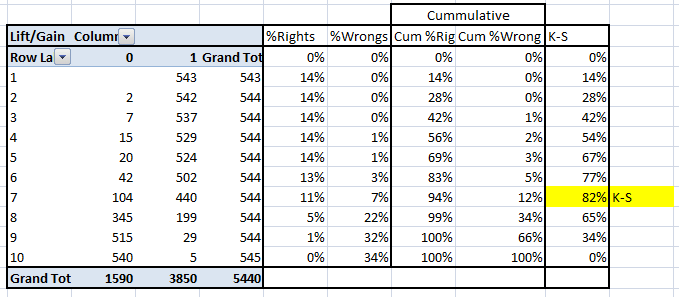We can also plot the %Cumulative Good and Bad to see the maximum separation. Following is a sample plot :The metrics covered till here are mostly used in classification problems. Till here, we learnt about confusion matrix, lift and gain chart and kolmogorov-smirnov chart. Let’s proceed and learn few more important metrics.

## 5. Area Under the ROC curve (AUC – ROC)

This is again one of the popular metrics used in the industry.  The biggest advantage of using ROC curve is that it is independent of the change in proportion of responders. This statement will get clearer in the following sections.

Let’s first try to understand what is ROC (Receiver operating characteristic) curve. If we look at the confusion matrix below, we observe that for a probabilistic model, we get different value for each metric.Hence, for each sensitivity, we get a different specificity.The two vary as follows: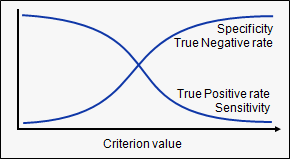The ROC curve is the plot between sensitivity and (1- specificity). (1- specificity) is also known as false positive rate and sensitivity is also known as True Positive rate. Following is the ROC curve for the case in hand.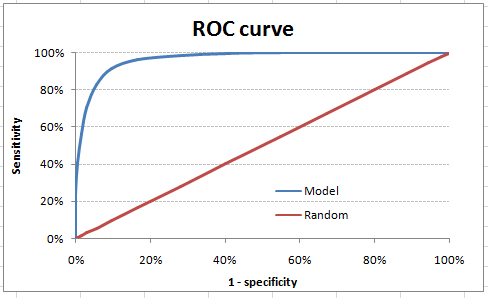Let’s take an example of threshold = 0.5 (refer to confusion matrix). Here is the confusion matrix :As you can see, the sensitivity at this threshold is 99.6% and the (1-specificity) is ~60%. This coordinate becomes on point in our ROC curve. To bring this curve down to a single number, we find the area under this curve (AUC).

Note that the area of entire square is 1*1 = 1. Hence AUC itself is the ratio under the curve and the total area. For the case in hand, we get AUC ROC as 96.4%. Following are a few thumb rules:

• .90-1 = excellent (A)
• .80-.90 = good (B)
• .70-.80 = fair (C)
• .60-.70 = poor (D)
• .50-.60 = fail (F)

We see that we fall under the excellent band for the current model. But this might simply be over-fitting. In such cases it becomes very important to to in-time and out-of-time validations.

Points to Remember:

1. For a model which gives class as output, will be represented as a single point in ROC plot.

2. Such models cannot be compared with each other as the judgement needs to be taken on a single metric and not using multiple metrics. For instance, model with parameters (0.2,0.8) and model with parameter (0.8,0.2) can be coming out of the same model, hence these metrics should not be directly compared.

3. In case of probabilistic model, we were fortunate enough to get a single number which was AUC-ROC. But still, we need to look at the entire curve to make conclusive decisions. It is also possible that one model performs better in some region and other performs better in other.

Why should you use ROC and not metrics like lift curve?

Lift is dependent on total response rate of the population. Hence, if the response rate of the population changes, the same model will give a different lift chart. A solution to this concern can be true lift chart (finding the ratio of lift and perfect model lift at each decile). But such ratio rarely makes sense for the business.

ROC curve on the other hand is almost independent of the response rate. This is because it has the two axis coming out from columnar calculations of confusion matrix. The numerator and denominator of both x and y axis will change on similar scale in case of response rate shift.

## 6. Log Loss

AUC ROC considers the predicted probabilities for determining our model’s performance. However, there is an issue with AUC ROC, it only takes into account the order of probabilities and hence it does not take into account the model’s capability to predict higher probability for samples more likely to be positive. In that case, we could us the log loss which is nothing but negative average of the log of corrected predicted probabilities for each instance.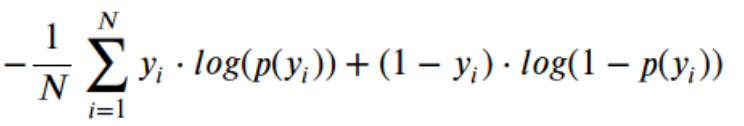• p(yi) is predicted probability of positive class
• 1-p(yi) is predicted probability of negative class
• yi = 1 for positive class and 0 for negative class (actual values)

Let us calculate log loss for a few random values to get the gist of the above mathematical function:

Logloss(1, 0.1) = 2.303

Logloss(1, 0.5) = 0.693

Logloss(1, 0.9) = 0.105

If we plot this relationship, we will get a curve as follows: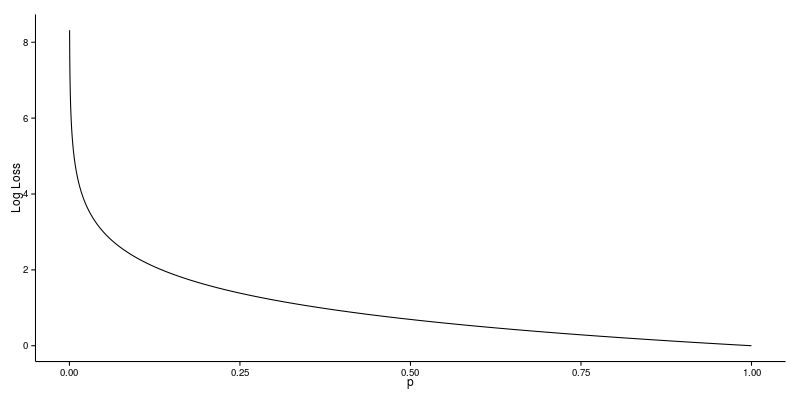It’s apparent from the gentle downward slope towards the right that the Log Loss gradually declines as the predicted probability improves. Moving in the opposite direction though, the Log Loss ramps up very rapidly as the predicted probability approaches 0.

So, lower the log loss, better the model. However, there is no absolute measure on a good log loss and it is use-case/application dependent.

Whereas the AUC is computed with regards to binary classification with a varying decision threshold, log loss actually takes “certainty” of classification into account.

## 7. Gini Coefficient

Gini coefficient is sometimes used in classification problems. Gini coefficient can be straigh away derived from the AUC ROC number. Gini is nothing but ratio between area between the ROC curve and the diagnol line & the area of the above triangle. Following is the formulae used :

Gini = 2*AUC – 1

Gini above 60% is a good model. For the case in hand we get Gini as 92.7%.

## 8. Concordant – Discordant ratio

This is again one of the most important metric for any classification predictions problem. To understand this let’s assume we have 3 students who have some likelihood to pass this year. Following are our predictions :

A – 0.9

B – 0.5

C – 0.3

Now picture this. if we were to fetch pairs of two from these three student, how many pairs will we have? We will have 3 pairs : AB , BC, CA. Now, after the year ends we saw that A and C passed this year while B failed. No, we choose all the pairs where we will find one responder and other non-responder. How many such pairs do we have?

We have two pairs AB and BC. Now for each of the 2 pairs, the concordant pair is where the probability of responder was higher than non-responder. Whereas discordant pair is where the vice-versa holds true. In case both the probabilities were equal, we say its a tie. Let’s see what happens in our case :

AB  – Concordant

BC – Discordant

Hence, we have 50% of concordant cases in this example. Concordant ratio of more than 60% is considered to be a good model. This metric generally is not used when deciding how many customer to target etc. It is primarily used to access the model’s predictive power. For decisions like how many to target are again taken by KS / Lift charts.

## 9. Root Mean Squared Error (RMSE)

RMSE is the most popular evaluation metric used in regression problems. It follows an assumption that error are unbiased and follow a normal distribution. Here are the key points to consider on RMSE:

1. The power of ‘square root’  empowers this metric to show large number deviations.
2. The ‘squared’ nature of this metric helps to deliver more robust results which prevents cancelling the positive and negative error values. In other words, this metric aptly displays the plausible magnitude of error term.
3. It avoids the use of absolute error values which is highly undesirable in mathematical calculations.
4. When we have more samples, reconstructing the error distribution using RMSE is considered to be more reliable.
5. RMSE is highly affected by outlier values. Hence, make sure you’ve removed outliers from your data set prior to using this metric.
6. As compared to mean absolute error, RMSE gives higher weightage and punishes large errors.

RMSE metric is given by: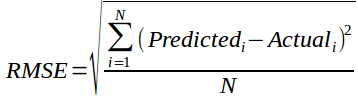where, N is Total Number of Observations.

## 10. Root Mean Squared Logarithmic Error

In case of Root mean squared logarithmic error, we take the log of the predictions and actual values. So basically, what changes are the variance that we are measuring. RMSLE is usually used when we don’t want to penalize huge differences in the predicted and the actual values when both predicted and true values are huge numbers.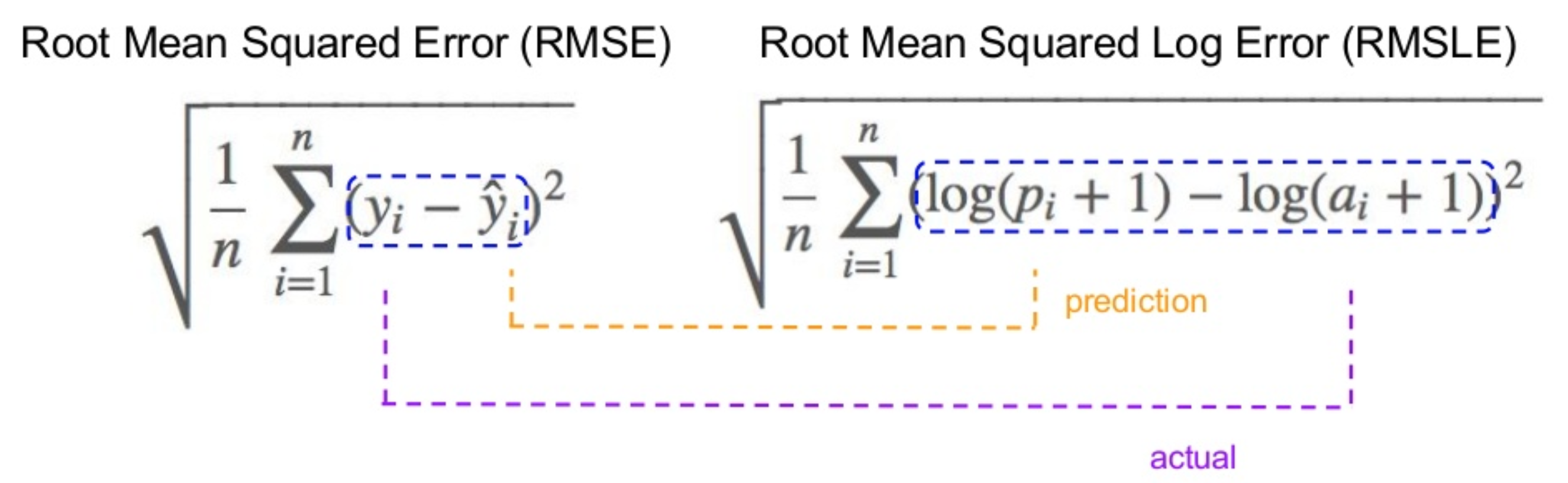1. If both predicted and actual values are small: RMSE and RMSLE are same.
2. If either predicted or the actual value is big: RMSE > RMSLE
3. If both predicted and actual values are big: RMSE > RMSLE (RMSLE becomes almost negligible)

We learned that when the RMSE decreases, the model’s performance will improve. But these values alone are not intuitive.

In the case of a classification problem, if the model has an accuracy of 0.8, we could gauge how good our model is against a random model, which has an accuracy of  0.5. So the random model can be treated as a benchmark. But when we talk about the RMSE metrics, we do not have a benchmark to compare.

This is where we can use R-Squared metric. The formula for R-Squared is as follows: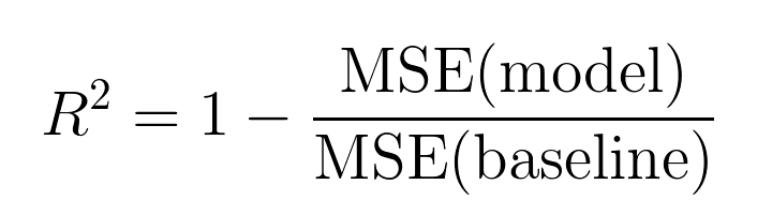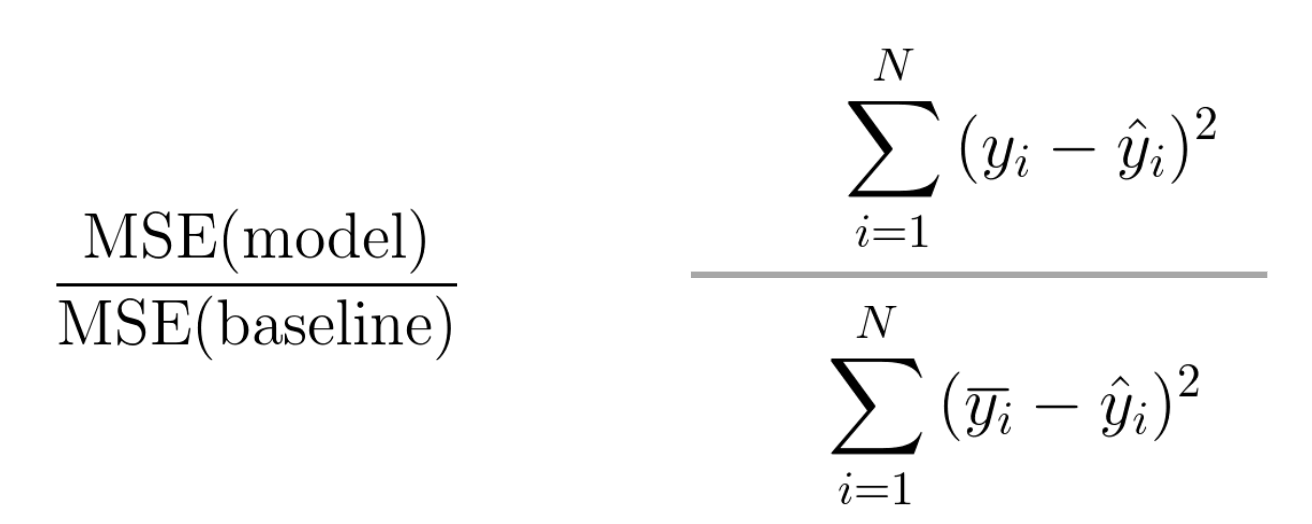MSE(model): Mean Squared Error of the predictions against the actual values

MSE(baseline): Mean Squared Error of  mean prediction against the actual values

In other words how good our regression model as compared to a very simple model that just predicts the mean value of target from the train set as predictions.

A model performing equal to baseline would give R-Squared as 0. Better the model, higher the r2 value. The best model with all correct predictions would give R-Squared as 1. However, on adding new features to the model, the R-Squared value either increases or remains the same. R-Squared does not penalize for adding features that add no value to the model. So an improved version over the R-Squared is the adjusted R-Squared. The formula for adjusted R-Squared is given by: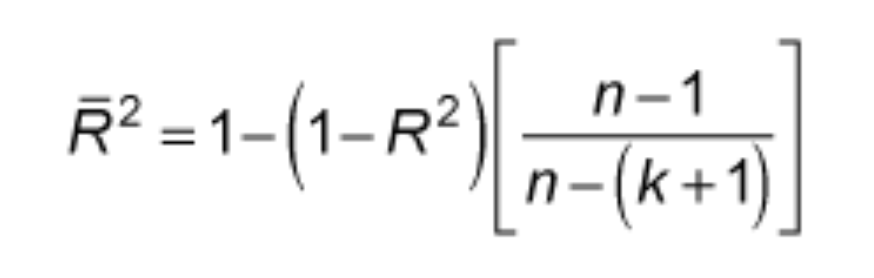k: number of features

n: number of samples

As you can see, this metric takes the number of features into account. When we add more features, the term in the denominator n-(k +1) decreases, so the whole expression increases.

If R-Squared does not increase, that means the feature added isn’t valuable for our model. So overall we subtract a greater value from 1 and adjusted r2, in turn, would decrease.

Beyond these 11 metrics, there is another method to check the model performance. These 7 methods are statistically prominent in data science. But, with arrival of machine learning, we are now blessed with more robust methods of model selection. Yes! I’m talking about Cross Validation.

Though, cross validation isn’t a really an evaluation metric which is used openly to communicate model accuracy. But, the result of cross validation provides good enough intuitive result to generalize the performance of a model.

Let’s now understand cross validation in detail.

## 12. Cross Validation

Let’s first understand the importance of cross validation. Due to busy schedules, these days I don’t get much time to participate in data science competitions. Long time back, I participated in TFI Competition on Kaggle. Without delving into my competition performance, I would like to show you the dissimilarity between my public and private leaderboard score.

### Here is an example of scoring on Kaggle!

For TFI competition, following were three of my solution and scores (Lesser the better) :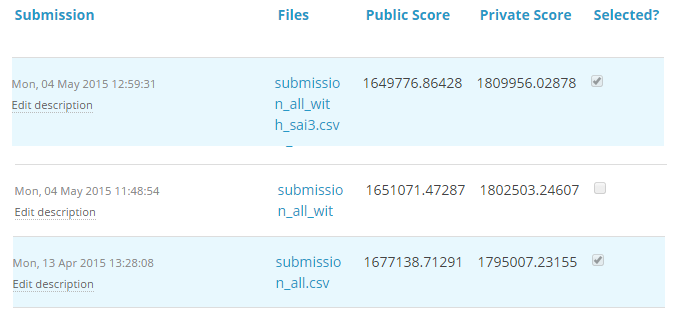You will notice that the third entry which has the worst Public score turned to be the best model on Private ranking. There were more than 20 models above the “submission_all.csv”, but I still chose “submission_all.csv” as my final entry (which really worked out well). What caused this phenomenon ? The dissimilarity in my public and private leaderboard is caused by over-fitting.

Over-fitting is nothing but when you model become highly complex that it starts capturing noise also. This ‘noise’ adds no value to model, but only inaccuracy.

In the following section, I will discuss how you can know if a solution is an over-fit or not before we actually know the test results.

### The concept : Cross Validation

Cross Validation is one of the most important concepts in any type of data modelling. It simply says, try to leave a sample on which you do not train the model and test the model on this sample before finalizing the model.Above diagram shows how to validate model with in-time sample. We simply divide the population into 2 samples, and build model on one sample. Rest of the population is used for in-time validation.

Could there be a negative side of the above approach?

I believe, a negative side of this approach is that we loose a good amount of data from training the model. Hence, the model is very high bias. And this won’t give best estimate for the coefficients. So what’s the next best option?

What if, we make a 50:50 split of training population and the train on first 50 and validate on rest 50. Then, we train on the other 50, test on first 50. This way we train the model on the entire population, however on 50% in one go. This reduces bias because of sample selection to some extent but gives a smaller sample to train the model on. This approach is known as 2-fold cross validation.

### k-fold Cross validation

Let’s extrapolate the last example to k-fold from 2-fold cross validation. Now, we will try to visualize how does a k-fold validation work.This is a 7-fold cross validation.

Here’s what goes on behind the scene : we divide the entire population into 7 equal samples. Now we train models on 6 samples (Green boxes) and validate on 1 sample (grey box). Then, at the second iteration we train the model with a different sample held as validation. In 7 iterations, we have basically built model on each sample and held each of them as validation. This is a way to reduce the selection bias and reduce the variance in prediction power. Once we have all the 7 models, we take average of the error terms to find which of the models is best.

### How does this help to find best (non over-fit) model?

k-fold cross validation is widely used to check whether a model is an overfit or not. If the performance metrics at each of the k times modelling are close to each other and the mean of metric is highest. In a Kaggle competition, you might rely more on the cross validation score and not on the Kaggle public score. This way you will be sure that the Public score is not just by chance.

### How do we implement k-fold with any model?

Coding k-fold in R and Python are very similar. Here is how you code a k-fold in Python :

``from sklearn import cross_validation model = RandomForestClassifier(n_estimators=100)` `#Simple K-Fold cross validation. 5 folds.` `#(Note: in older scikit-learn versions the "n_folds" argument is named "k".)` `cv = cross_validation.KFold(len(train), n_folds=5, indices=False)` `results = []` `# "model" can be replaced by your model object # "Error_function" can be replaced by the error function of your analysis` `for traincv, testcv in cv:` `            probas = model.fit(train[traincv], target[traincv]).predict_proba(train[testcv])` `            results.append( Error_function )` `#print out the mean of the cross-validated results` `print "Results: " + str( np.array(results).mean() )``

### But how do we choose k?

This is the tricky part. We have a trade off to choose k.

For a small k, we have a higher selection bias but low variance in the performances.

For a large k, we have a small selection bias but high variance in the performances.

Think of extreme cases :

k = 2  : We have only 2 samples similar to our 50-50 example. Here we build model only on 50% of the population each time. But as the validation is a significant population, the variance of validation performance is minimal.

k = number of observations (n) :  This is also known as “Leave one out”. We have n samples and modelling repeated n number of times leaving only one observation out for cross validation. Hence, the selection bias is minimal but the variance of validation performance is very large.

Generally a value of k = 10 is recommended for most purpose.

## End Notes

Measuring the performance on training sample is point less. And leaving a in-time validation batch aside is a waste of data. K-Fold gives us a way to use every singe datapoint which can reduce this selection bias to a good extent. Also, K-fold cross validation can be used with any modelling technique.

In addition, the metrics covered in this article are some of the most used metrics of evaluation in a classification and regression problems.

Which metric do you often use in classification and regression problem ? Have you used k-fold cross validation before for any kind of analysis? Did you see any significant benefits against using a batch  validation? Do let us know your thoughts about this guide in the comments section below.

### You want to apply your analytical skills and test your potential? Then participate in our Hackathons and compete with Top Data Scientists from all over the world.

You can also read this article on Analytics Vidhya's Android APP•Vishwa says:

I think adding multilogloss , in this would be useful. It is a good matrix to identify better model in case of multi class classification.

•venugopal says:

Very useful

•Mario says:

Hi,

Great post thanks. Just in number one confusion matrix you miscalculated the negative predicted value.
It is not 1.7% but 98.3%. Same for specificity (59.81% instead of 40.19%). Since you reuse this example for ROC, your curve is actually better. But anyways your argument still holds. Nicely presented.

•venkata ramana reddy kandula says:

Its a good information

•Sanjay.S says:

Very informative and useful article.Thank you..

•Tamara says:

Considering provided confusion matrix, negative predicrive value is 951/967 or not? Is there an error in confusion matrix example or in formulas?

•Amogh says:

Yes I agree with Tamara the negative predictive value should be 98.3454%. Can you please confirm Tavish

•Rishabh0709 says:

Hi Tavish,
Thanks again , for this valuable article.
It would be great , if along with this very informative explanation , you can also provide how to code it , preferably in R.
Thanks.

•Mac Lover says:

Introduction p Predictive Modeling works on constructive feedback principle. You build a model. Get feedback from metrics, make improvements and …

•Sudheer says:

Excellent article! thanks for the effort.

•Sudheer says:

Excellent article! Thanks a lot!!

•Sudhindra says:

Can we all the list of Statistical Models and the application scenarios please for a novice person like me?

•Jorge says:

Are there metrics for non supervised models as k-means for example? Thanks!

•Pranav Dar says:

Hi Jorge,

We have updated the article with evaluation metrics for unsupervised learning as well.

•Scott Bradshaw says:

Hi, can you further explain this “Lift is dependent on total response rate of the population”? Is this only applicable when you are able to correctly predict 100% of the 1st (or more) deciles?